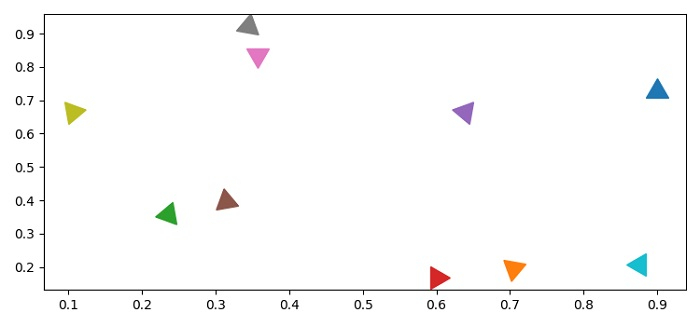# Is it possible to control Matplotlib marker orientation?

To control matplotlib marker orientation, we can use marker tuple that contains a number of sides, style and rotation or orientation of the marker.

## Steps

• Set the figure size and adjust the padding between and around the subplots.

• Create x and y data points using numpy.

• Make an array of 10 different rotations.

• Zip x, y and i. Iterate them and plot the points using plot() method with marker tuple.

• To display the figure, use show() method.

## Example

import numpy as np
from matplotlib import pyplot as plt
plt.rcParams["figure.figsize"] = [7.50, 3.50]
plt.rcParams["figure.autolayout"] = True
x = np.random.rand(10)
y = np.random.rand(10)
i = np.linspace(0, 10, 10)
for x, y, i in zip(x, y, i):
plt.plot(x, y, marker=(3, 0, i*45), linestyle='None', markersize=20)
plt.show()

## Output